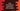# Java Arrays binarySearch method explanation with example## java.util.Arrays class binarySearch method explanation with examples:

java.util.Arrays class provides different methods to use with arrays. binarySearch method is used to search for an element by using binary search algorithm in different types of arrays.

In this post, we will learn the definition of binarySearch method and how to use binarySearch method with different examples.

## Definition of binarySearch:

The binarySearch method is defined as like below:

``````public static <T> int binarySearch(T[] arr,
int from,
int to,
T element,
Comparator<? super T> c)``````

Here,

• arr is the array to search for the element using binary search.
• c is the comparator used to sort the array. The array must be sorted in ascending order before we call this method. For an unsorted array, it will return undefined. Also, if the array contains multiple elements equal to the element we are searching for, it might return any value, there is no guarantee on it. If a null value is passed to it, it will use natural ordering.
• from is the index from where the search should be started. This value is inclusive. It is an optional value. If we don’t provide its value, the search will start from the first element of the array.
• to is the index where the search should stop. This value is exclusive. It is an optional value. If we don’t provide its value, the search will end at the end of the array.
• element is the value to find in the array.

### Return value of binarySearch:

It returns the index of the element if it is in the array. Else, it returns -(insertion point) - 1. Here, insertion point is:

• the index of the first element in the array which is greater than the element.
• to if it is given or last index, i.e. the end index and all elements are less than the element we are searching for.

### Exceptions:

It might throw any of the following three exceptions:

#### ClassCastException:

This is thrown if the elements are not comparable using the comparator or search element is not comparable with other elements in the array by using the given comparator.

#### IllegalArgumentException:

It is thrown if the to index is less than the from index, to < from

#### ArrayIndexOutOfBoundsException:

This exception is thrown if from < 0 or to > array length

#### byte array:

``````binarySearch(byte[] arr, byte element)
static int	binarySearch(byte[] arr, int from, int to, byte element)``````

It searches for element in the byte array arr using binary search algorithm.

#### char array:

``````binarySearch(char[] arr, char element)
static int	binarySearch(char[] arr, int from, int to, char element)``````

It searches for element in the character array arr using binary search algorithm.

#### double array:

``````binarySearch(double[] arr, double element)
static int	binarySearch(double[] arr, int from, int to, double element)``````

It searches for element in the double array arr using binary search algorithm.

#### float array:

``````binarySearch(float[] arr, float element)
static int	binarySearch(float[] arr, int from, int to, float element)``````

It searches for element in the float array arr using binary search algorithm.

#### int array:

``````binarySearch(int[] arr, int element)
static int	binarySearch(int[] arr, int from, int to, int element)``````

It searches for element in the int array arr using the binary search algorithm.

#### long array:

``````binarySearch(long[] arr, long element)
static int	binarySearch(long[] arr, int from, int to, long element)``````

It searches for element in the long array arr using the binary search algorithm.

#### Object array:

``````binarySearch(Object[] arr, Object element)
static int	binarySearch(Object[] arr, int from, int to, Object element)``````

It searches for element in the Object array arr using the binary search algorithm.

#### short array:

``````binarySearch(short[] arr, short element)
static int	binarySearch(short[] arr, int from, int to, short element)``````

It searches for element in the short array arr using the binary search algorithm.

### Example of binarySearch:

Let’s try it with an example:

``````import java.util.Arrays;

public class Main {

public static void main(String[] args) {
int[] intArray = {1, 2, 3, 4, 5, 6, 7, 11, 18, 21};

System.out.println("Searching for 1: " + Arrays.binarySearch(intArray, 1));
System.out.println("Searching for 10: " + Arrays.binarySearch(intArray, 10));
System.out.println("Searching for 22: " + Arrays.binarySearch(intArray, 22));
}
}``````

It will print the below output:

``````Searching for 1: 0
Searching for 10: -8
Searching for 22: -11``````

Here, we are using binarySearch to search for an element in an integer array.

• intArray is an array of integers.
• The first one prints 0 because the position of 1 is 0, i.e. the index of 1 in the array.
• The second one prints -8 because, we don’t have 10 in the array and the place for 10 is after 7, which will be at index 7. So, it will print -7-1 i.e. -8
• The last statement prints -11, because 22 is not in the array and if we have to place it, we will place it at the end of the array. The index will be 10. So it is -10 - 1 or -11.